Courses

# Test: Boolean Operations

## 30 Questions MCQ Test RRB JE for Computer Science Engineering | Test: Boolean Operations

Description
This mock test of Test: Boolean Operations for Computer Science Engineering (CSE) helps you for every Computer Science Engineering (CSE) entrance exam. This contains 30 Multiple Choice Questions for Computer Science Engineering (CSE) Test: Boolean Operations (mcq) to study with solutions a complete question bank. The solved questions answers in this Test: Boolean Operations quiz give you a good mix of easy questions and tough questions. Computer Science Engineering (CSE) students definitely take this Test: Boolean Operations exercise for a better result in the exam. You can find other Test: Boolean Operations extra questions, long questions & short questions for Computer Science Engineering (CSE) on EduRev as well by searching above.
QUESTION: 1

### Consider a carry look-ahead adder for adding two n-bit integers, built using gates of fan-in at most two. The time to perform addition using this adder is:

Solution:

Look-ahead carry generator gives output in constant time if the fan in = number of inputs.

For example, it will take  O(1) to calculate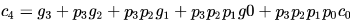, if the OR gate with 5 inputs is present.
If we have 8 inputs, and OR gate with 2 inputs, to build an OR gate with 8 inputs, we will need 4 gates in level-1, 2 in level-2 and 1 in level-3. Hence 3 gate delays, for each level.
Similarly, an n-input gate constructed with 2-input gates, the total delay will be O(log n).

QUESTION: 2

### Consider an eight-bit ripple-carry adder for computing the sum of A and B, where A and B are integers represented in 's complement form. If the decimal value of A is one, the decimal value of B that leads to the longest latency for the sum to stabilize is ___________

Solution:

in case of -1  we get bit sequence 11111111 adding this we get a carry upto carry flag,so largest time to ripple !

QUESTION: 3

### An N-bit carry lookahead adder, where N is a multiple of 4, employs ICs 74181 (4 bit ALU) and 74182 ( 4 bit carry lookahead generator). The minimum addition time using the best architecture for this adder is

Solution:

For N = 64 bits
Suppose you want to build a 64 bit adder then you need 16 4-bit ALU and 16 4-bit carry generator, at this point there will be 16 carries that will ripple through these 16 ALU modules, to speed up the adder we need to get rid of these 16 rippling carries, now we can again use 4 4-bit carry generator to generate these 16 carries, now we have only 4 carries to ripple through, again we can use the same trick to minimize the rippling of these 4 carries, we can use an additional 4-bit carry generator which will generate these carry and we are done :) there will be no more propagation of carry among the ALU modules.
So the we have used 3 level of 4-bit carry generator, and the time taken to add 64 bits will be proportional to 3 which is log464.
So in general to add N-bits it takes Log4N time.

QUESTION: 4

The number of full and half-adders required to add 16-bit numbers is:

Solution:

QUESTION: 5

A 4-bit carry look ahead adder, which adds two 4-bit numbers, is designed using AND, OR, NOT, NAND, NOR gates only.Assuming that all the inputs are available in both complemented and uncomplemented forms and the delay of each gate is one time unit, what is the overall propagation delay of the adder? Assume that the carry network has been implemented using two-level AND-OR logic.

Solution:

It would take 6 time units.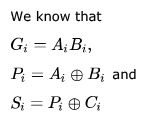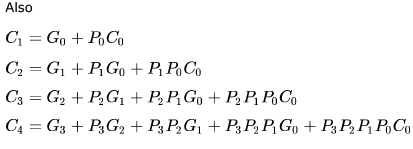XOR can be implemented in 2 levels; level-1 ANDs and Level-2 OR. Hence it would take 2 time units to calculate  Pi and Si
The 4-bit addition will be calculated in 3 stages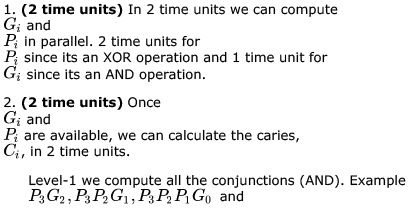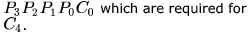Level-2 we get the carries by computing the disjunction (OR).
3. (2 time units) Finally we compute the Sum in 2 time units, as its an XOR operation.
Hence the total is 2 + 2 + 2 = 6 time units.

QUESTION: 6

The maximum gate delay for any output to appear in an array multiplier for multiplying two-bit numbers is:

Solution:

In a N X M array multiplier we have N * M AND gates and (M-1) N bit adders used.
Total delay in N X M (N>=M) array multiplier due to AND gate in partial products at all level is just 1 unit AND gate delay as the operation is done parallel wise at each step. Now delays at level 1 to (M-1) is = (M-1)*delay due to 1 unit of N bit adder.  Therefore the maximum gate delay is O(M) but here M=N therefore O(N).

QUESTION: 7

Consider an array multiplier for multiplying two n bit numbers. If each gate in the circuit has a unit delay, the total delay of the multiplier is:

Solution:

Take A = A1 A2 A3 A4
B =   B1 B2 B3 B4

Now to multiply these two numbers.
1 and gate require b1 multiply with a1 a2 a3 a4.
1 and gate require b2 multiply with a1 a2 a3 a4.
1 and gate require b3 multiply with a1 a2 a3 a4.
1 and gate require b4 multiply with a1 a2 a3 a4.
Now 3 or gate require.
Total 7 gates are required for 4 bit take n bit u find 2n-1.
So time complexity will be = ϴ(n)

QUESTION: 8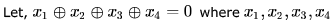are Boolean variables,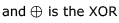operator.

Which one of the following must always be TRUE?

Solution: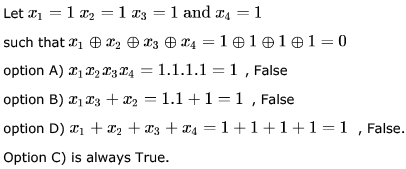QUESTION: 9

The total number of Boolean functions which can be realised with four variables is:

Solution:

A Boolean function of 4 variables is a function from a set 24 = 16 of elements (all combinations of 4 variables) to a set of 2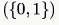elements. So, number of such functions will be 216 = 65,536

QUESTION: 10

The simultaneous equations on the Boolean variables x, y, z and w,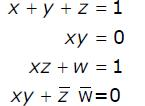have the following solution for x, y, z and w, respectively:

Solution:

Statement 1 :  x + y + z = 1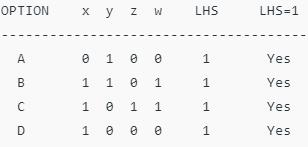Till now, all the options are possible.

Statement 2 : x y = 0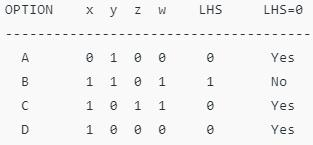Since LHS ≠ 0, B is not possible.

Statement 3 : x z + w = 1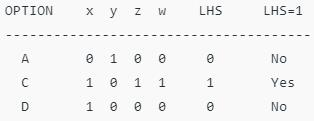Since LHS ≠ 1, A and D are not possible.

Statement 4 : x y + z’ w’ = 0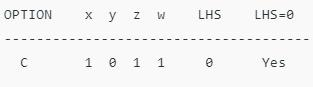Thus, C is the correct option.

QUESTION: 11

Consider the Boolean operator # with the following properties :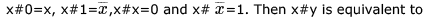Solution:

These are properties of XOR function.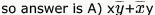QUESTION: 12

Simplify Y = AB’ + (A’ + B)C

Solution:

Y = AB’ + (A’ + B)C = AB’ + (A’ + B)C = AB’ + (AB’)’C = AB’ + C.

QUESTION: 13

Find values of Boolean variables A,B,C which satisfy the following equations:

• A+ B = 1
• AC = BC
• A + C = 1
• AB = 0
Solution:

From A + B = 1 and AB = 0
We get either of A,b is 1 and another is 0.
Now AC = BC ,here C has to be 0 (because A,b  has different values)
C = 0
Now A + C = 1
So, A = 1 and Ab = 0, So,  B = 0
So we get
A = 1, B = 0 , C = 0
These are the values.

QUESTION: 14

Complement of the expression A’B + CD’ is:

Solution:

(A’B + CD’)’ = (A’B)'(CD’)’ = (A” + B’)(C’ + D”) = (A + B’)(C’ + D).

QUESTION: 15

In boolean algebra, the OR operation is performed by which properties?

Solution:

The expression for Associative property is given by A+(B+C) = (A+B)+C & A*(B*C) = (A*B)*C.
The expression for Commutative property is given by A+B = B+A & A*B = B*A.
The expression for Distributive property is given by A+BC=(A+B)(A+C) & A(B+C) = AB+AC.

QUESTION: 16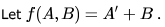Simplified expression for function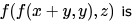Solution: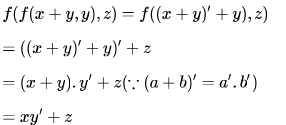QUESTION: 17

A Boolean function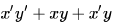Solution:

x'y' + x'y = x'(y+y') = x'
x' + xy = x' + y

QUESTION: 18

The function AB’C + A’BC + ABC’ + A’B’C +  AB’C’ is equivalent to

Solution: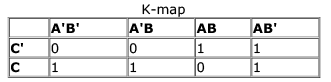So, the equivalent expression will be A'C + AC' + AB'

QUESTION: 19

Which of the following expressions is equivalent to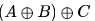Solution: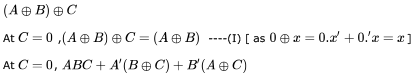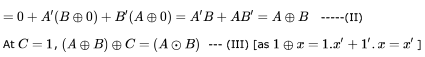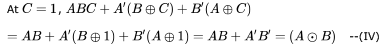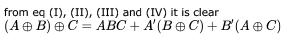QUESTION: 20

Define the connective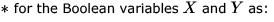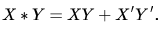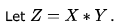Consider the following expressions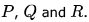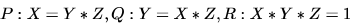Which of the following is TRUE?

Solution:

P:
Y * Z = Y * (X*Y)
= Y * (XY + X'Y')
= Y (XY + X'Y') + Y' (XY + X'Y')'
= XY + Y' ((X' + Y') (X+ Y))
= XY + Y' (X'Y + XY')
= XY + XY'
= X(Y + Y')
= X
So, P is valid.
Q:
X * Z = X * (X*Y)
= X * (XY + X'Y')
= X (XY + X'Y') + X' (XY + X'Y')'
= XY + X' ((X' + Y') (X+ Y))
= XY + X' (X'Y + XY')
= XY + X'Y
= Y(X + X')
= Y
So, Q is also valid.
R:
X * Y * Z = (X * Y) * (X * Y)
= (XY + X'Y') * (XY + X'Y')
= (XY + X'Y') (XY + X'Y') + (XY + X'Y')' (XY + X'Y')'
= (XY + X'Y') + (XY + X'Y')' (Since, AA = A)  = 1 (Since A + A' = 1)
So, R is also valid.
Hence, D choice.

QUESTION: 21

If P,Q,R are Boolean variables, then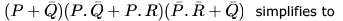Solution: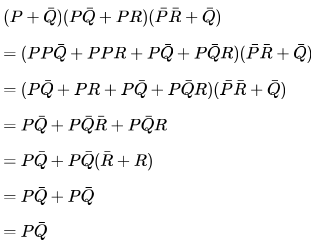QUESTION: 22

In boolean algebra, the OR operation is performed by which properties?

Solution:

The expression for Associative property is given by A + (B + C) = (A + B) + C & A * (B * C) = (A * B) * C.
The expression for Commutative property is given by A + B = B + A & A * B = B * A.
The expression for Distributive property is given by A + BC = (A + B)(A + C) & A(B + C) = AB + AC.

QUESTION: 23

Which one of the following expressions does NOT represent exclusive NOR of x and y?

Solution:

A: means both are either true OR both are false. then it will be true = ExNOR
B & C: Whenever any one of the literal is complemented then ExOR can be turned to ExNOR and complement sign on the literal can be removed. So these two also represents ExNOR operation of x and y.
Hence, the correct answer is option D it is the ExOR operation b/w the two.

QUESTION: 24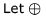denote the exclusive OR (XOR) operation. Let '1' and '0' denote the binary constants. Consider the following Boolean expression for F over two variables P and Q:
The equivalent expression for  is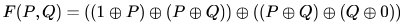The equivalent expression for F is

Solution:

XOR is associative and commutative. Also,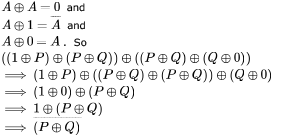QUESTION: 25

If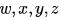are Boolean variables, then which one of the following is INCORRECT?

Solution:

Option C (wx'(y+xz')+w'.x')y = (wx'y+wx'xz'+w'x')y  =
xx' = 0.
So
Wx'y+w'x'y = x'y(w+w') = x'y

QUESTION: 26

Choose the correct alternatives (more than one may be correct) and write the corresponding letters only: The operation which is commutative but not associative is:

Solution:
1. Every logic gate follows Commutative law.
2. AND, OR, Ex-OR, EX-NOR follows Associative law also. NAND, NOR doesn’t follow Associative law.
QUESTION: 27

Which of the following expressions is not equivalent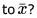Solution:

x OR 1 = 1 and so x NOR 1 = 0.

QUESTION: 28

When multiplicand Y is multiplied by multiplier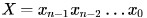using bit-pair recoding in Booth's algorithm, partial products are generated according to the following table.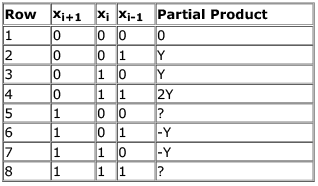The partial products for rows 5 and 8 are

Solution:

Partial product is calculated by using bit pair recording in booths algorithm, which is improvement technique used in booths algorithm. Here we consider 3 bits at a time for getting the partial product. This eliminates the worst case behaviour of normal Booth's algorithm.
"100" corresponds to -2M and "111" corresponds to 0 -2 X(i+1) + x (i) + X(i-1)

QUESTION: 29

Booth’s algorithm for integer multiplication gives worst performance when the multiplier pattern is

Solution:

The worst case of an implementation using Booth’s algorithm is when pairs of 01s or 10s occur very frequently in the multiplier.

QUESTION: 30

Booth's coding in 8 bits for the decimal number -57 is

Solution:

There are 2 ways for answering This questions.
Way 1 -> Convert 57 to Binary & Get 2's complement. It is "11000111" & Attach one extra 0 to right of it
110001110

To calculate booth code subtract right digit from left digit in every consecutive 2 digits.
So 11-> 0 , 10 -> +1, .. finally 10 -> +1